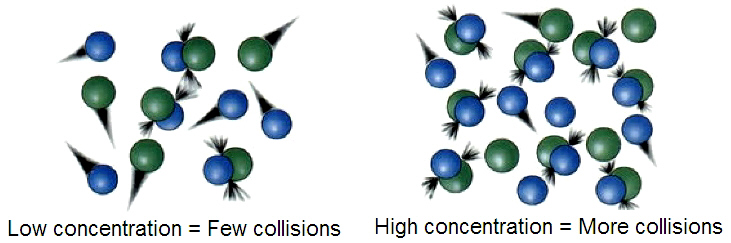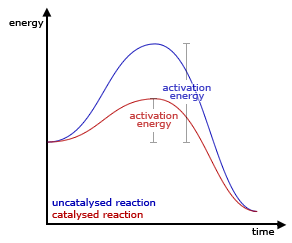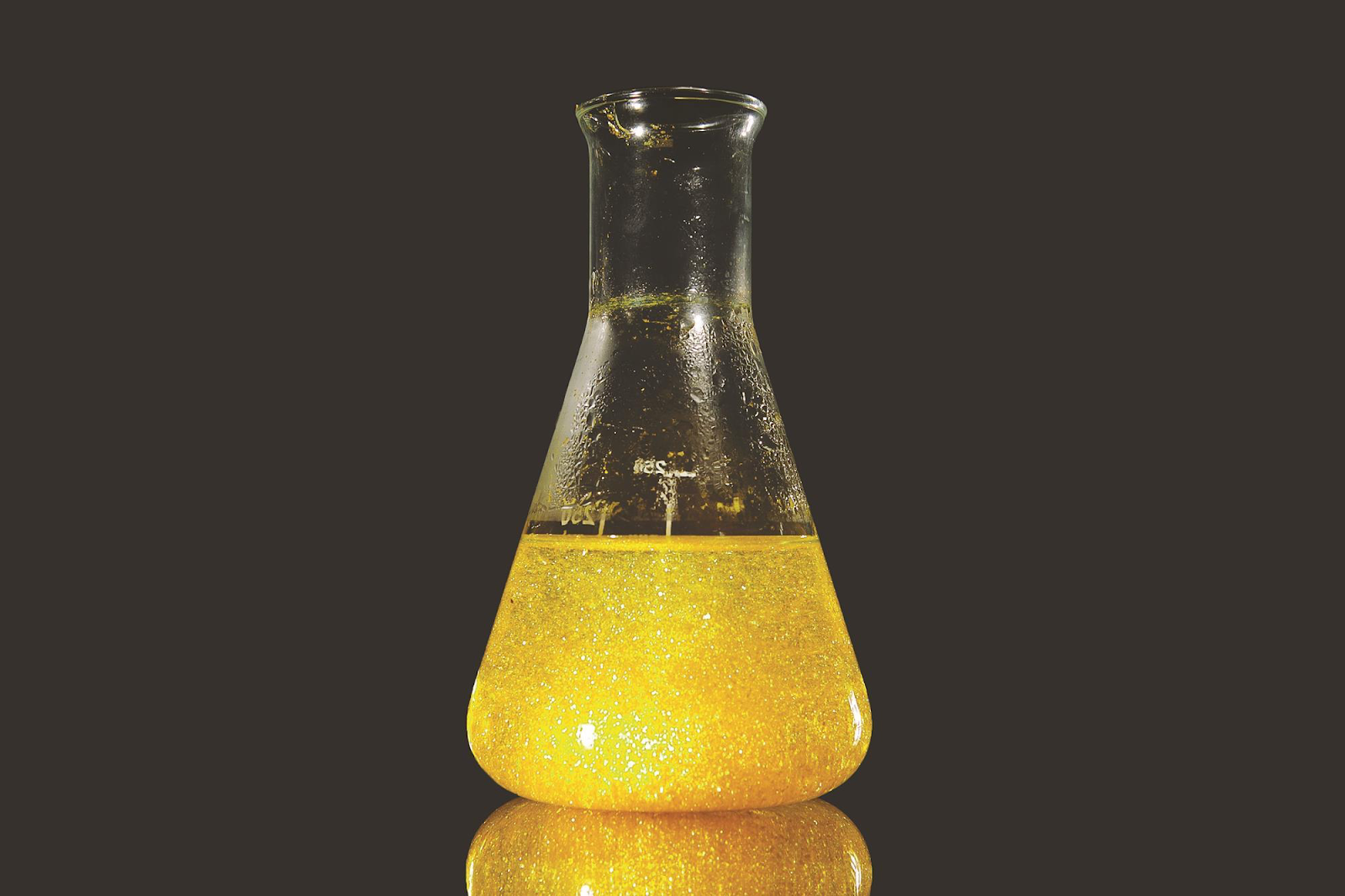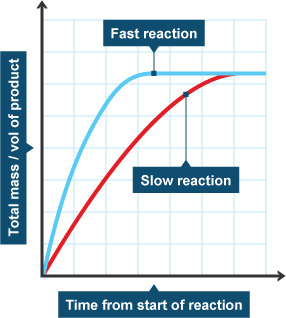# Rates of Reaction

## Rates of Reaction

What you need to know:

How different environmental factors can affect the rate of reaction.

The rate of a reaction is just how fast a reaction happens!

1. __Temperature __- the higher the temperature, higher the energy, the faster the particles collide, the more likely they are to collide and react, the faster the rate of reaction
2. __Concentration __- The more concentrated a reactant is, the closer the particles are. If the particles are closer together they are more likely to collide and react with one another. Higher concentration increases the rate of reaction.
3. __Catalysts __- Catalysts provide an alternative route for the reactants. This alternative route requires less energy, therefore catalysts increase the rate of reaction.
4. Surface area - The larger the surface area, the more particles are exposed and are more likely to react. The larger the surface area the faster the rate of reaction.

If these don’t quite make sense, look at the next section and come back!

## Collision Theory

What you need to know:

What collision theory is.

What are the limitations of collision theory (how is it different to what actually happens in real life).

1. Collision theory is just a way in which we explain how particles react. It’s not ACTUALLY what happens (since what actually happens is very very complicated), but it’s good enough.
2. In collision theory, we describe particles as spheresthat are all whizzing around. How fast they move depends on how much energy they have (so the hotter they are, the faster they move). When two particles collide at high speeds, the two react!
3. Two big things to know about collision theory are:

The more the particles collide, the more likely they are to react. This increases the rate of reaction.

Particles have to collide with enough energy in order to react with one another.## Catalysts

What you need to know:

What a catalyst is.

How a catalyst works, in terms of activation energy.

A catalyst is a substance that is added to a reaction to speed up the reaction. The catalyst is not changed or used up in the reaction.

The type of catalyst needed depends on the reaction, but they all work in very similar ways.

1. They provide an alternative reaction route which requires less energy - the minimum energy the particles need in order to react is called the activation energy. Catalysts decrease this reaction energy so that reactions happen easier and quicker!Biological catalysts (catalysts used in our bodies) are called enzymes. These help chemical reactions happen in our bodies. Without them we would not be able to survive since the chemical reactions wouldn’t occur as much as we need them to.

## Measuring Rates of Reaction

What you need to know:

How can we measure the rate of reaction of different types of reactions.Whether you use the amount of reactant used or the amount of product formed depends on what the reaction actually is.

For example:

1. If a precipitate (solid) is formed, you can measure how much has been formed
2. This can be done by putting the flask on a piece of paper with an x drawn on. You can time how long it takes for you to not be able to see through to the x anymore (because of the precipitate build up)
3. For some experiments, you can filter off the precipitate and weight it after a certain amount of time.1. If a precipitate (solid) is formed, you can measure how much has been formed
2. This can be done by putting the flask on a piece of paper with an x drawn on. You can time how long it takes for you to not be able to see through to the x anymore (because of the precipitate build up)
3. For some experiments, you can filter off the precipitate and weight it after a certain amount of time.

## Calculating Rates of Reaction

What you need to know:

How to calculate the rate of reaction by using a reaction graph.There are two main ways to find the rate of a reaction, using the equation above or finding the gradient of a reaction rate graph.The gradient ‘slope’ of the graph tells you how fast the reaction is. The steeper the gradient, the faster the reaction! For this to work you need to make sure that time is on the x-axis.

Since the reaction rate is changing all the time, we need to pick a time to work out the reaction rate.

As you can see on the graph above, the two lines stop at the same place, this is because they both create the same amount of product. However, the blue line got there first! This is because the blue line has a steeper gradient (a faster reaction rate).